MORE SITES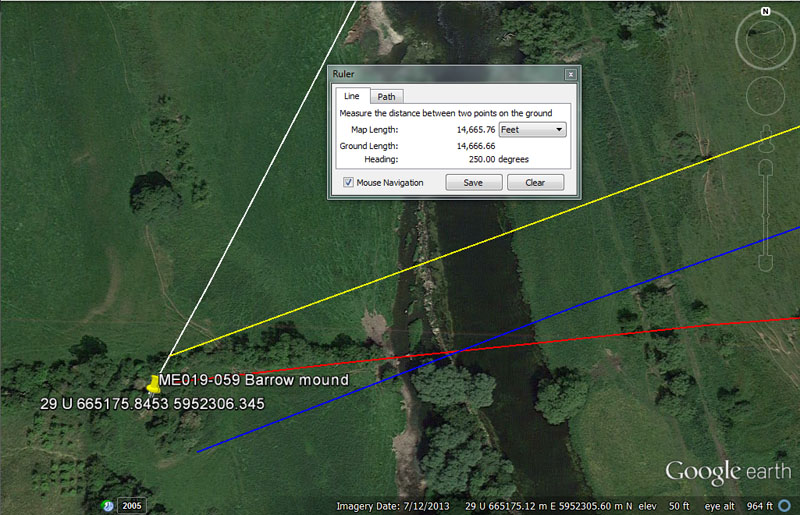Many of the archaeological sites on the County Meath register give no outwards signs of ever having been there. Some that existed within living memory are marked on old maps. Indications that yet others once existed show up after field crops are harvested and crop marks of former sites are temporarily visible seasonally in the newly denuded fields. This barrow mound site at Rossnaree was destroyed in the 1940s.

From Knowth = 3250’ @ 207.36-degrees.

The distance is in mnemonic reference to a "13" mathematical progression, useful in tracking the solar component of the Sabbatical Calendar. The value 3.25 is 1/4th of 13.

The angle is, of course, navigational and 2073.6-miles would be 1/6th of the 24883.2-mile equatorial circumference.

From Newgrange = 4950’ @ 264-degrees.

The distance code is navigational and 4950-miles would be 1/5th of the 24750-mile equatorial circumference.

The angle is in the same genre and 2640' would be half a mile of 5280' , whereas 26400' would be 1/4950th of the 24750-mile equatorial circumference.

From Dowth Mound = 11000’ @ 69.12-degrees.

This distance would equate to 2/3rds of a league (16500').

The angle is, of course navigational and, under the 24883.2-mile assignment, 69.12-miles would be 1-degree of equatorial arc.

From Dowth Henge = 14666.6666’ @ 250 - 70-degrees.

This distance value would equate to 2 & 7/9ths miles of 5280' or 1/36th of 100-miles. It would also be 1/8910th of the 24750-mile equatorial circumference.

The two-way degree angle codes are self-explanatory.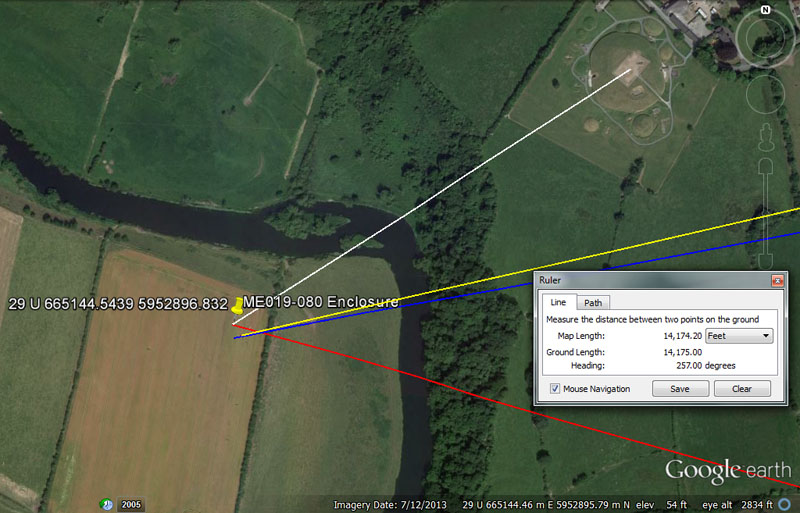Although now only a seasonally visible crop mark, a large enclosure once sat at this position in this Rossnaree field.

From Knowth = 1814.4’ @ 237.6-degrees.

This coded distance is navigational and 181440' would be half of the 362880' in 1-degree of arc under the 24883.2-mile (of 5250') equatorial circumference. Alternatively, 1814.4' would be 18-seconds (100.8') of arc.

The degree angle is also navigational and is part of the 19008 mathematical progression, explained in relation to site ME019-049001. Also. a distance of 23760' would amount to 4.5-miles of 5280' and, at the same time 1/5500th of the 24750-mile equatorial circumference.

From Newgrange = 5156.25’ @ 105.6-degrees.

This distance is navigational and would equate to 3000 Egyptian Royal Cubits of 20.625". This distance is also 1/10th the length from Dowth chambered mound to the Mound of the Hostages atop the Hill of Tara.

The degree angle is navigational coding and 105.6' would be 1/50th of the 5280'-mile.

From Dowth Mound = 10560’ @ 259.2 – 79.2-degrees.

The sum of 10560' equates to 2-miles of 5280' each.

The outwards running angle of 259.2-degrees is in homage to the 25920-year duration of the Precession of the Equinoxes. Reading this as the return angle of 79.2-degrees is in mnemonic reference to the 7920-mile diameter of the Earth.

From Dowth Henge = 14175’ @ 77-degrees.

This distance is 2.7-miles of 5250' or 22.5 stadia.

The 77-degrees angle is self explanatory as an "11" series number and 77 X 77 & 1/7th = 5940' or 1 ancient Scottish mile.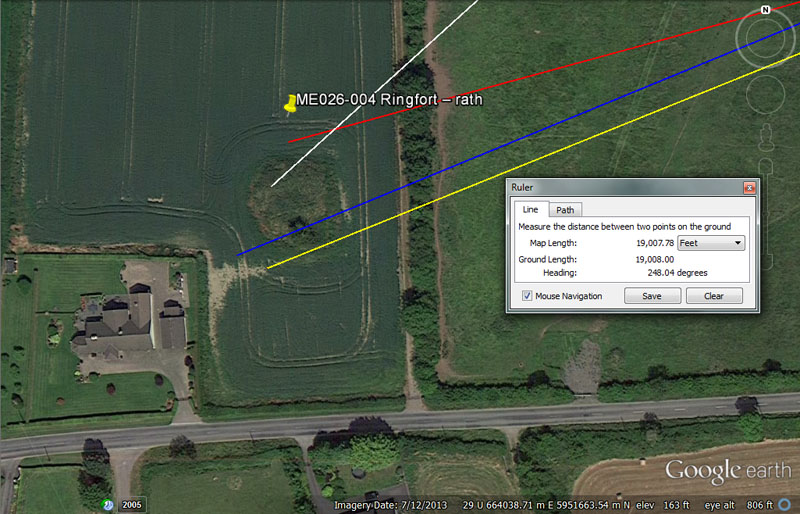This very impressive Ringfort rath is also found at Rossnaree and the effort expended in its construction attests to its dynamic positional codes.

From Knowth = 7200’ @ 47.52-degrees.

The distance provides a value that is multi-use and, amongst many other things, 720-miles would be 1/11th of the 7920-mile diameter of the Earth. Also, this distance equates to half of a geomancer's mile of 14400'.

The degree angle is also navigational and a distance of 47520' would be 9-miles of 5280' or 8-miles of 5940'. It would also be 1/2750th of the 24750-mile equatorial circumference.

From Newgrange = 9072’ @ 74.25-degrees.

This distance is navigational and 9072' equates to 1.5-minutes of equatorial arc. The Great Pyramid @ 756' per side = 9072".

The degree angle is also navigational and a distance of 742.5' would equate to 1/8th of the ancient Scottish mile @ 5940', whereas 7425' would be 1.25 Scottish miles.

From Dowth Mound = 15360’ @ 247.5-degrees.

This distance is navigational coding and 153.6-miles would be 1/162nd of the 24883.2-mile equatorial circumference and 1.536-miles would be 1/45th of 1-degree of arc.

The degree angle is, of course, in homage to the 24750-mile equatorial circumference.

From Dowth Henge = 19008’ @ 68.04-degrees.

The distance is navigational and equates to 3.6-miles of 5280' or 3.2-miles of 5940.

The degree angle is in homage to the 6804-day lunar nutation cycle.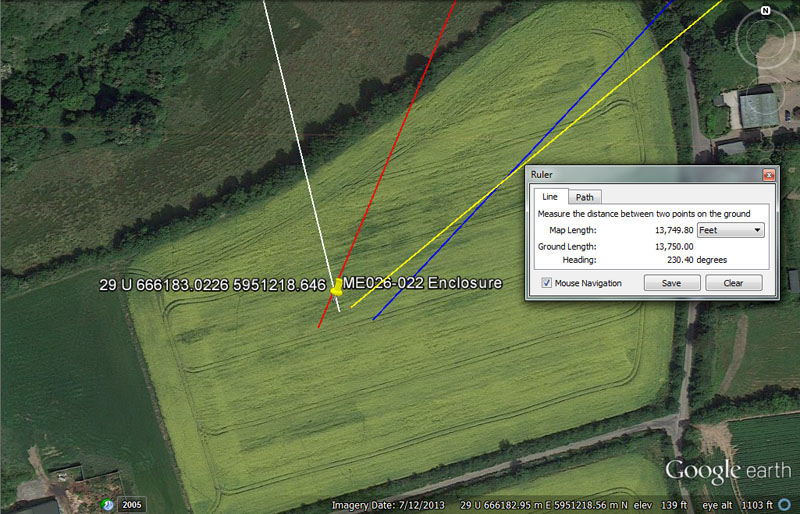Again, within this Gilltown field there was an enclosure, now reduced to a seasonally visible crop mark The archaeological register places it at exactly this position shown.

From Knowth = 6804’ @ 345.6-degrees.

Again, this distance for students issuing forth from Knowth chambered mound is in homage to the 6804-day lunar nutation cycle.

The degree angle of travel is navigational and 345.6-miles would be 1/72nd of the 24883.2-mile equatorial circumference or 5-degrees of arc.

From Newgrange = 4608’ @ 22.5-degrees.

The distance is navigational and 460.8-miles would be 1/54th of the 24883.2-mile equatorial circumference.

The angle of 22.5-degrees is self-explanatory as 1/16th of 360-degrees.

From Dowth Mound 10368’ @ 43.2-degrees.

This distance encodes the speed at which the 24883.2-mile around Earth rotates in 24-hours or 1036.8 MPH.

The degree angle value is multi-functional and can be applied to many lunar or navigational calculations. For example, 432' would equate to 1/14th of 6048' (1-minute of equatorial arc). The Great Pyramid (9072" per side) equates to 432 Hebrew or Celtic cubits of 21" each. The sum of 43.2-miles would be 1/576th of the 24883.2-mile equatorial circumference. The 6804-day lunar nutation cycle would be 15.75 periods of 432-days (10368-hours), etc.

From Dowth Henge = 13750’ @ 50.4-degrees.

This length is navigational coding and 13.75-miles would be 1/5th of 1-degree of arc (68.75-miles) under the 24750-mile navigational method. Alternatively, the sum of 1375-miles would be 1/18th of the 24750-mile circumference and 13750' would be 1/9504th of the distance around the world. Remember: 95040' would be 18 miles of 5280' or 16-miles of 5940').

The degree angle is navigational as well as lunar and 50.4' would equate to half of the 100.8' that constitutes 1-second of arc under the 24883.2-mile (of 5250') equatorial circumference. Likewise, there would be 135 intervals of 50.4-days in the 6804-day lunar nutation cycle.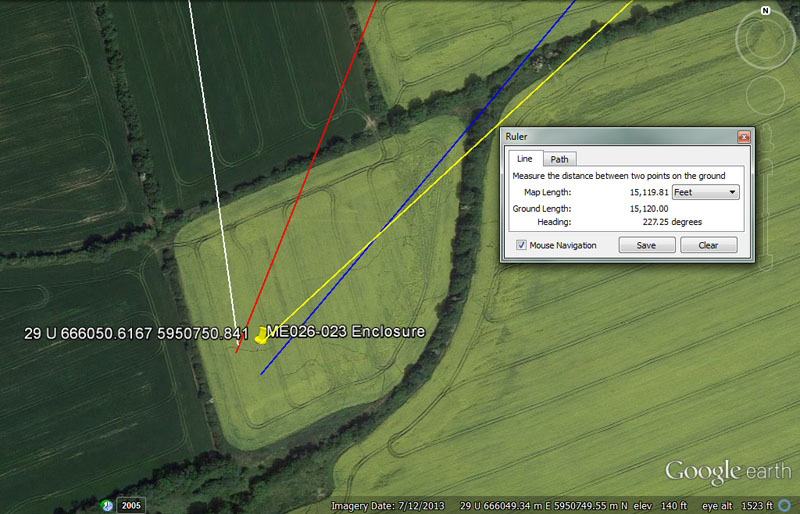And yet another all-but-erased enclosure, reduced to a crop mark, in yet another field at Gilltown.

From Knowth = 8167.5’ @ 171.875-degrees.

The distance is navigational and 81675' would equate to 1/1600th of the 24750-mile equatorial circumference. A distance of 81675' would be 13,75 ancient Scottish miles of 5940'.

The degree angle is navigational and 17.1875-miles (17 & 3/16ths) would be 1/4th of 1-degree of arc (68.75-miles) under the 24883.2-mile assignment.

From Newgrange = 6180.34’ @ 201.6-degrees.

The fact that ancient savants were able to calculate this number and recognise its significance is extraordinary. The value is based upon the PHI reciprocal and was of profound importance to the cousin civilisations for squaring the circle and fabricating round tubs of perfect cubic inch capacity for the market places. The circular bases of round tubs could achieve perfect square inch values if the diameters were in increments of 6.18034". For the ancient English bushel, this meant a circular base of 3 X 6.18034" to achieve 270 square inches, with sides extending 8" above the base to achieve a capacity of 2160 cubic inches (lunar and Precession of the Equinoxes coding).

The degree angle is, of course, coding 1/30th of 1-minute of arc.

From Dowth Mound = 11880’ @ 220-degrees.

This distance is 2 ancient Scottish miles of 5940' or 2.25-miles of 5280'.

The angle is self-explanatory as an "11" series number and 220' would be 1/24th of 5280'.

From Dowth Henge = 15120' @ 47.25-degrees.

Again, a repeat of coding 2.5-minutes of equatorial arc in this length.

The degree angle is lunar and the Khafre Pyramid had a design height of 472.5' (4 X 118.125' or, in days 4 lunar months). Also, the Khafre Pyramid was 15/16ths the length of the Great Pyramid. Therefore, 756 ÷ 16 = 47.25' ... X 15 = 708.75' (or, in days, 2-lunar years of 354.375-days each).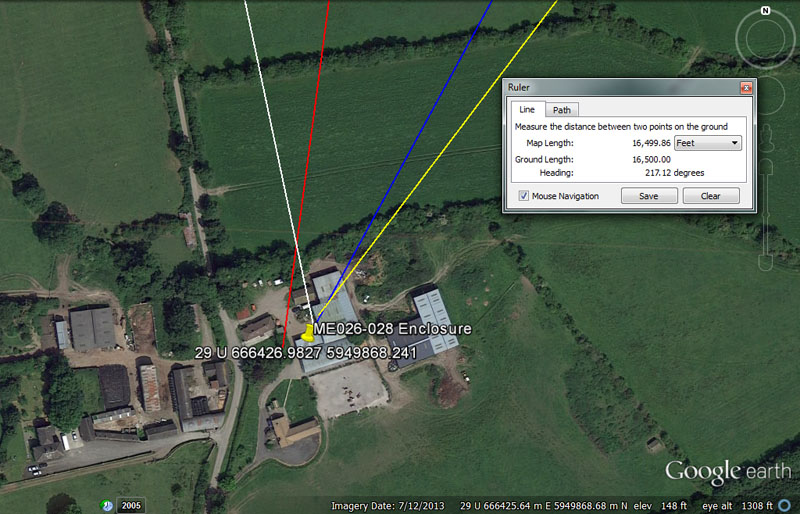Further to the East at Lougher an enclosure was built-over by farm houses and outbuildings. The archaeological register places it at exactly these coordinates.

From Knowth = 11250’ @ 168-degrees.

The length is navigational and the value 112.5-degrees would be 5/16ths of 360-degrees. In the navigational reading of the Earth's circumference as 24750-miles, the sum of 1125-miles would be 1/22nd part.

The degree angle code is multi-functional and 1680' would be 1/4th of the 6720' Irish mile. Likewise, 168' would be 1/36th of 6048' (1-minute of arc). In the 6804-day lunar nutation cycle there would be 40.5 intervals of 168-days. There are 168-hours in a week of 7-days, etc.

From Newgrange = 8750’ @ 187.5-degrees.

A length of 8750' is 1 & 2/3rds miles of 5250' or 875' is 1/6th of 5250'. At the end of a 7-year Sabbatical Calendar count 8.75 intercalary days had to be added to fully correct the solar count, etc.

The degree angle is navigational and 187.5' would be 1/28th of the 5250' mile adopted by the late era Greeks. Also, in the 24750-mile reading of the equatorial circumference, the sum of 187.5-miles would be 1/132nd part.

From Dowth Mound = 13608’ @ 28.53-degrees.

This distance is 6804' X 2 and is in homage to the 6804-day lunar nutation cycle.

The degree angle is lunar and the Khafre Pyramid (Egypt's Pyramid of the Moon) was 2835' for all 4 sides, or, in days, 8 lunar years of 354.375-days each.

From Dowth Henge = 16500’ @ 37.125-degrees.

This distance from Dowth Henge is 1 league or 3.125-miles of 5280'.

The degree angle is navigational and 371.25' would be 1/16th of the 5940' Scottish mile. There would be 6 & 2/3rds X 3712.5-miles in the 24750-mile reading.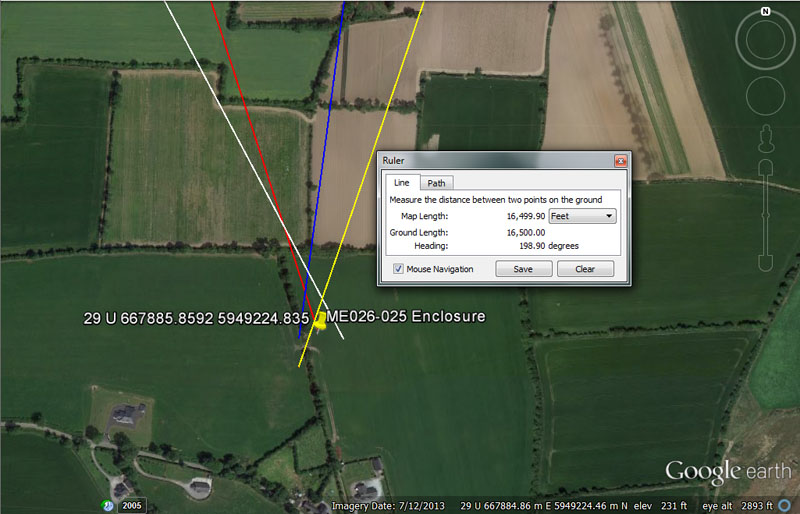The archaeological register places another enclosure at this point of a Gillinstown field.

From Knowth = 15120’ @ 151.875-degrees.

Again a repeat of the 2.5-minutes of arc reading or 1/10th the distance from the centre of Dowth Henge to the trig cairn atop Slieve Gullion Mountain in County Armagh.

The degree angle is navigational and 151.875' (151 & 7/8ths) would be 1/32nd of the 4860' mile adopted by the Romans.

From Newgrange = 11520’ @ 161.8034-degrees.

This length is navigational coding and 115.2-miles would equate to 1/216th of the 24883.2 circumference. At Stonehenge the outer rim of the Sarsen Circle achieved 345.6' of circumference or 11.52' per lintel.

The degree angle is in homage to the PHI ratio of 1.618034 to 1, which, like an understanding of the PI ratio of 3.1416 to 1, is essential to the concept of civilisation.

From Dowth Mound = 14400’ @ 187.5-degrees.

This distance is 1 geomancer's mile. The Egyptian pyramid acre was 28800 square feet or 14400 X 2. The distance of 14400' would equate to 1/9072nd of the 24883.2-mile (of 5250') equatorial circumference.

Again we have a repeat expression of 187.5-degrees and this value in feet is 1/28th of the 5250' mile or, in the 24750-mile reading of the equatorial circumference, the sum of 187.5-miles would be 1/132nd part.

From Dowth Henge = 16500’ @ 18.9-degrees.

Again, this site sits 1-league from Dowth Henge.

The angle code is navigational and the Great Pyramid is 189' X 4 per side. Also, 189' would be 1/32nd of 1-minute of arc in the Earth's circumference under the 5250'-mile navigational method.

Continue